# Line Integrals

## Calculus 3### 143 Practice Problems

06:41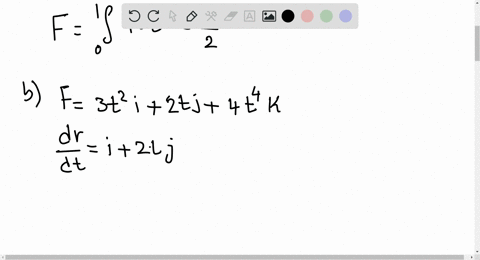Thomas Calculus 12

In Exercises $7-12,$ find the line integrals of $F$ from $(0,0,0)$ to $(1,1,1)$ over each of the following paths in the accompanying figure. a. The straight-line path $C_{1} : \mathbf{r}(t)=t \mathbf{i}+t \mathbf{j}+t \mathbf{k}, \quad 0 \leq t \leq 1$ b. The curved path $C_{2} : \mathbf{r}(t)=t \mathbf{i}+t^{2} \mathbf{j}+t^{4} \mathbf{k}, \quad 0 \leq t \leq 1$ c. The path $C_{3} \cup C_{4}$ consisting of the line segment from $(0,0,0)$ to $(1,1,0)$ followed by the segment from $(1,1,0)$ to $(1,1,1)$
$$\mathbf{F}=3 y \mathbf{i}+2 x \mathbf{j}+4 z \mathbf{k}$$

Integration in Vector Fields
Vector Fields and Line Integrals: Work, Circulation, and Flux04:06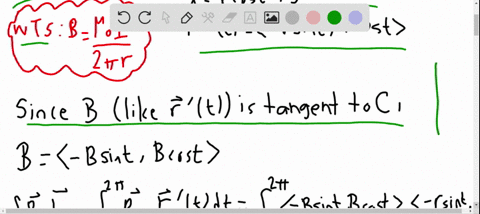Essential Calculus Early Transcendentals

Experiments show that a steady current $I$ in a long wire produces a magnetic field $\mathbf{B}$ that is tangent to any circle that lies in the plane perpendicular to the wire and whose
center is the axis of the wire (as in the figure at the right). Ampere's Law relates the electric current to its magnetic effects and states that
$$\int_{C} \mathbf{B} \cdot d \mathbf{r}=\mu_{0} I$$
where $I$ is the net current that passes through any surface bounded by a closed curve $C,$ and $\mu_{0}$ is a constant called the permeability of free space. By taking $C$ to be a circle
with radius $r,$ show that the magnitude $B=|\mathbf{B}|$ of the magnetic field at a distance $r$ from the center of the wire is $$B=\frac{\mu_{0} I}{2 \pi r}$$

VECTOR CALCULUS
Line Integrals04:35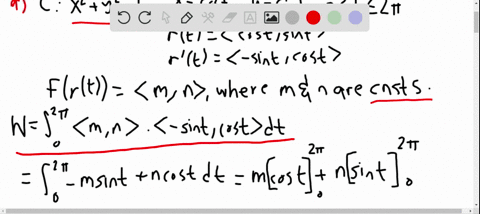Essential Calculus Early Transcendentals

(a) Show that a constant force field does zero work on a particle that moves once uniformly around the circle $x^{2}+y^{2}=1 .$
(b) Is this also true for a force field $\mathbf{F}(\mathbf{x})=k \mathbf{x},$ where $k$ is a constant and $\mathbf{x}=\langle x, y\rangle ?$

VECTOR CALCULUS
Line Integrals04:49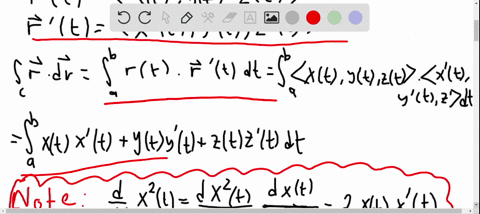Essential Calculus Early Transcendentals

If $C$ is a smooth curve given by a vector function $\mathbf{r}(t),$ $a \leqslant t \leqslant b,$ show that $$\int_{C} \mathbf{r} \cdot d \mathbf{r}=\frac{1}{2}\left[|\mathbf{r}(b)|^{2}-|\mathbf{r}(a)|^{2}\right]$$

VECTOR CALCULUS
Line Integrals04:07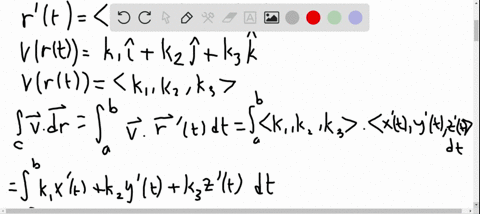Essential Calculus Early Transcendentals

If $C$ is a smooth curve given by a vector function $\mathbf{r}(t)$ $a \leqslant t \leqslant b,$ and $\mathbf{v}$ is a constant vector, show that
$$\int_{C} \mathbf{v} \cdot d \mathbf{r}=\mathbf{v} \cdot[\mathbf{r}(b)-\mathbf{r}(a)]$$

VECTOR CALCULUS
Line Integrals03:34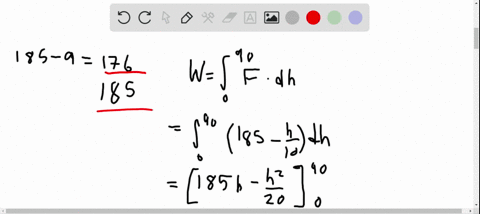Essential Calculus Early Transcendentals

Suppose there is a hole in the can of paint in Exercise 43 and 9 lb of paint leaks steadily out of the can during the man's ascent. How much work is done?

VECTOR CALCULUS
Line Integrals03:10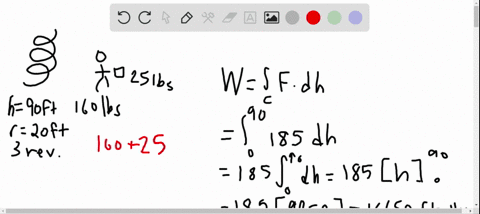Essential Calculus Early Transcendentals

A 160 -lb man carries a $25-$ lb can of paint up a helical staircase that encircles a silo with a radius of 20 $\mathrm{ft}$ . If the silo is 90 $\mathrm{ft}$ high and the man makes exactly three complete revolutions climbing to the top, how much work is done by the man against gravity?

VECTOR CALCULUS
Line Integrals05:43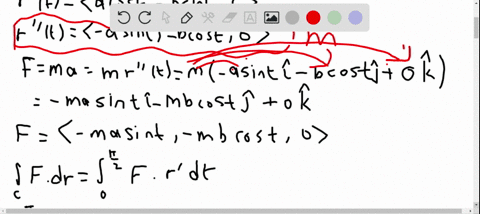Essential Calculus Early Transcendentals

An object with mass $m$ moves with position function
$$\mathbf{r}(t)=a \sin t \mathbf{i}+b \cos t \mathbf{j}+c t \mathbf{k} \quad 0 \leqslant t \leqslant \pi / 2$$
Find the work done on the object during this time period.

VECTOR CALCULUS
Line Integrals03:24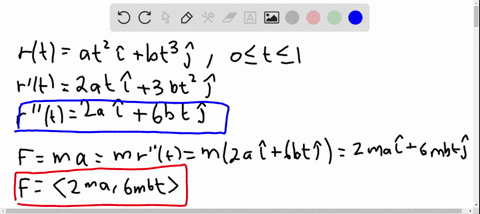Essential Calculus Early Transcendentals

The position of an object with mass $m$ at time $t$ is
$\mathbf{r}(t)=a t t^{2} \mathbf{i}+b t^{3} \mathbf{j}, 0 \leqslant t \leqslant 1$
(a) What is the force acting on the object at time $t ?$
(b) What is the work done by the force during the time
interval 0$\leqslant t \leqslant 1 ?$

VECTOR CALCULUS
Line Integrals07:23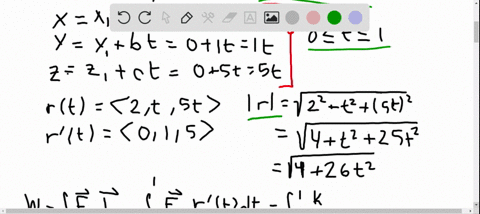Essential Calculus Early Transcendentals

The force exerted by an electric charge at the origin on a charged particle at a point $(x, y, z)$ with position vector $\mathbf{r}=\langle x, y, z\rangle$ is $\mathbf{F}(\mathbf{r})=K \mathbf{r} /|\mathbf{r}|^{3}$ where $K$ is a constant.
(See Example 5 in Section $13.1 .$ ) Find the work done as the particle moves along a straight line from (2,0,0) to (2,1,5)

VECTOR CALCULUS
Line Integrals05:38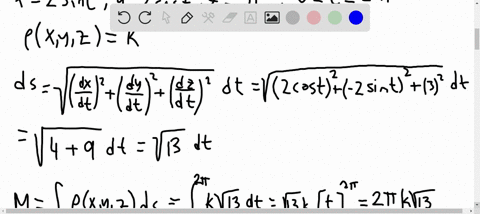Essential Calculus Early Transcendentals

(a) Write the formulas similar to Equations 4 for the center of mass $(\overline{x}, \overline{y}, \overline{z})$ of a thin wire in the shape of a space curve $C$ if the wire has density function $\rho(x, y, z)$ . (b) Find the center of mass of a wire in the shape of the helix $x=2 \sin t, y=2 \cos t, z=3 t, 0 \leqslant t \leqslant 2 \pi,$ if the density is a constant $k$ .

VECTOR CALCULUS
Line Integrals07:12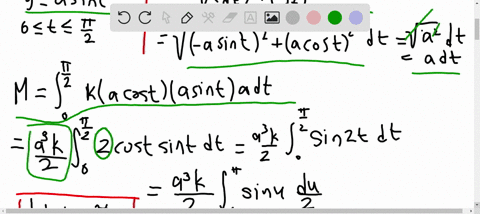Essential Calculus Early Transcendentals

A thin wire has the shape of the first-quadrant part of the circle with center the origin and radius $a$ . If the density function is $\rho(x, y)=k x y,$ find the mass and center of mass of the wire.

VECTOR CALCULUS
Line Integrals1 2 3 4 5 ... 12